×
Get Full Access to UTD - STAT 2332 - Study Guide
Get Full Access to UTD - STAT 2332 - Study Guide

×

UTD / Statistics / STAT 2332 / When do we use the z-test?

# When do we use the z-test? Description

##### Description: Covers ch 23-28
14 Pages 135 Views 1 Unlocks
Reviews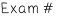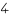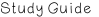## When do we use the z-test?Topics covered in Exam 4:

CH 23:

- The t-distribution

CH 26:

- Hypothesis Testing. The One-Sample z-Test

- Significance Value, P-value

CH 27:

- Two Sample Tests (Means)

NOTE: Sample Proportions Test is NOT covered in this study guide but may  possibly be on the exam.

CH 28:

- Chi-Square Test of Goodness of Fit

- Chi-Square Test of Independence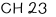## When do we use the t-distribution?If you want to learn more check out How do you determine if a change is statistically significant?

Confidence Interval estimate for �� when �� (standard deviation) is known: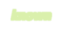��̅± �� ∙��√��

Where

��̅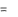average or mean of sample

## What is the matched pairs experiment?If you want to learn more check out What is an example of self­enhancement in the us?

�� = z-score

�� = standard deviation of population

�� = sample size

When we have normal distributions (bell curves), we use z-scores. z-score formula when we are using samples and �� is known:

�� =��̅− ��

��/√��

But sometimes we will get a situation where we don’t know ��. In this case, we  use “s” (t-distributions).

For the formula for finding s, look under highlighted “t-distribution” Confidence Interval estimate for �� when �� is unknown: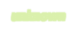Note: Assume population is normally distributed, �� is unknown, and sample size is  also small (< 25).

????Using t-distribution

��̅± ����−1,(1−��/2)��√��

Where

����−1,(1−��/2)is t-statistic with degrees of freedom value (can be found using t table with n-1 degrees of freedom) If you want to learn more check out What is an advantage for the south?

�� is given % confidence interval.

Ex: You have n=8 (degrees of freedom = 7) and 95% CI???? 1 − �� = 5% ???? 5/2= 2.5% ???? look at t-table with row n=7 and column labeled 2.5% or 0.025 ???? use t-statistic value 2.36

Confidence Interval estimate for �� when �� is unknown (non-normal) and sample  size is large (�� ≥ 25)

�� must be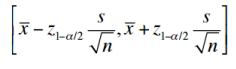within this

interval

Where

��1−��/2 is the upper 100(α/2)th percentile of the standard normal distribution  s = sample standard deviation (look for formula above) If you want to learn more check out Is tongue rolling homozygous or heterozygous?

�� = given % confidence interval

Sometimes, we’ll take two samples from two independent populations. Since we  have two populations, we’ll have two population means: ��1 and ��2. We want to  compare the two population means (��1 − ��2) using two samples. If you want to learn more check out What differentiates an acute from a persistent viral infection?

Ex: Compare SAT scores between males and females.

Confidence Interval for Difference of Population Means where (���� − ����): Chen, Min. “Chapter 23.” eLearning, 2016, pp. 10.

“v” = degrees of

freedom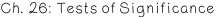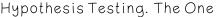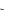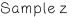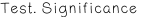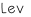Don't forget about the age old question of Who is louis xiv's great-grandson?

Tests of Significance: tests intending to assess the evidence provided by the data  in favor of some claim about a population parameter (��).

∙ Choose between 2 competing hypothesis:

Null hypothesis vs. Alternative hypothesis

Hypothesis: a statement about how true the population parameter (��) is Ex: ��: �� = 0 ���� ��: �� = 101 ���� ��: �� < 7 ���� ��: �� > 7 ���� ��: �� ≠ 180 Null Hypothesis ���� : hypothesis statement being tested. No effect or no

difference in population (�� = #) Ex: ��0: �� = 0 , ��0: �� = 140

or ��0

�� = ��0

- We either reject ���� or fail to reject ���� (more on this in later pages)

Alternative Hypothesis ���� (also written as H1) : the effect we suspect to  be true; this is the statement that we want to prove.

Ex: We suspect the actual mean to be more than 140.

����: �� > 140

This is a one-sided alternative hypothesis where

����: �� > ��0 (right sided alternative) or ����: �� < ��0 (left-sided

alternative)

Ex: We suspect the mean to not equal the null hypothesis of zero. ����: �� ≠ 0

This is a two-sided alternative hypothesis where

����: �� ≠ ��0

∙ If the null hypothesis is rejected, we accept the alternative hypothesis. We  decide on whether to reject or fail to reject ���� based on the p-value. P-value: probability that the observed outcome would occur if H0 is true; the  lower the p-value the stronger the evidence against H0.

- To find the p-value, you must calculate a z or t-statistic and look at a z or  t-table. Probability under the curve (shaded) is your p-value.

- Formulas to find the test statistic in later pages

- P-value of two sided test is twice as large as a one sided test. If the p-value is less than the alpha/significance value, reject null hypothesis. If the p is low reject the Ho.

For confidence intervals, if a test statistic or the value being tested is outside the  confidence interval, reject the H0. If we reject H0, accept/conclude Ha or H1 Otherwise, if we don’t reject the null hypothesis, then we “fail to reject H0”

z-test: used when:

∙ Population mean �� is unknown

∙ Population standard deviation �� is known

∙ Large n (≥25)

For one sample, use a one-sample z-test.

For two samples, use a two-sample z-test. (further explanation in later pages)

t-distribution: probability distribution shaped like a bell curve (normally  distributed) in which width/shape of the curve depends on the degrees of  freedom. Used when �� is unknown and sample size n is small (n < 25).

∙ degrees of freedom = �� − 1

∙ We replace the z-statistic with t-statistic, and �� with s

∙ If �� is unknown, substitute sample standard deviation “s” for ��.

�� = √1

��

�� − 1∑ (���� − ��̅)2

��=1

We use the t-distribution when we are using “s” and when sample sizes are  small.

Note: Student t-distribution is the same as t-distribution

As n increases???? t-distribution approaches a normal distribution (t ???? z) As n increases???? s decreases ???? s estimates �� more accurately As n increases???? degrees of freedom (n-1) increases???? bell curve is narrower

t-test: used when:

∙ Population mean is unknown

∙ Population standard deviation is unknown. Use “s” instead of �� ∙ Small n (<25).

For one sample, use a one sample t-test.

For two samples, use a two-sample t-test.

1. Determine if problem has one or two samples. State null and alternative  hypothesis.

2. Choose significance level (usually provided). If not stated, use �� = 0.05. 3. Calculate test statistic

For a one sample z-test:2 sample z-test:

�� =��̅− ��0

��/√��

p-value of 2 sided z-test = 1 − P(-# ≤ z ≤ #)

One sample t-test:

t , v = n – 1 �������������� ���� ����,1−��/2

2 sample t-test:

�� =(��1 − ��2) − (��1 − ��2)

√����2 (1��1+1��2), �� = ��1 + ��2 − 2

Degrees of

Freedom (v)

�������������� ���� ����,1−��

4. Use a z or t-table to find value of test statistic (this is your pvalue). 5. Compare value to significance level/alpha. If pvalue ≤ �� then reject H0,  conclude Ha. If pvalue > 0 then fail to reject H0 (H0 is true).

Type I Error: reject Ho, when is Ho is true.

Ex: build a restaurant when I shouldn’t have

Type II Error: fail to reject Ho, when Ha is true (Ho is false).

Ex: don’t build a restaurant when I should have

H0 is true

H0 is false

Reject H0

Type I Error

Correct decision

Don’t reject H0

Correct decision

Type II Error

This chapter will further explain the formulas from earlier pages, confidence  interval formulas, and proportions.

For Chi-square tests, skip to chapter 28.

- Recall that �� is mean of the population and �� is mean of a sample taken  from the population.

��1 − ��2 ���� �������������������������� ��ℎ�� �������� ���� ��1 − ��2

So the Expected Value (EV) of ��1 = ��1 and ��2 = ��2 , ��1 − ��2 = ��1 − ��2 - Variance is standard deviation squared (��2)

Variance of ��1 − ��2:

������(��1 − ��2) =��12

��1+��22

��2

Standard Error (SE) of two samples = square root of variance ����(��1 − ��2) = √��12

��1+��22

��2

- Distribution of ��1 − ��2 is approximately normal (bell-curve shape) if the  population is normal or by the central limit theorem (sample sizes are >30).

Recall that z-statistic of one sample is:

��/√���������������� − ����( �� )

�� =�� − ��

For 2 samples, z is:

����( �� )

valueExpected  value

�� =(��1 − ��2) − (��1 − ��2) √��12

��1+��22

��2

Standard error

However, the population variance ��2is usually unknown. Therefore, we must  use a t-statistic.

Recall that t-distributions use “s” (sample standard dev.) to approximate “��”

- The t-statistic uses ������(called the pooled variance estimator) instead of ��2 - For 2 samples of equal variance, t-statistic is:

�� =(��1 − ��2) − (��1 − ��2)

√����2 (1��1+1��2), �� = ��1 + ��2 − 2

Degrees of

Freedom (v)

�������������� ���� ����,1−��

Confidence Interval (CI) for ���� − ���� (equal variances)

(��1 − ��2) ± ����,1−��/2 × √����2 (1��1+1��2)

Notice how the above formulas are for equal variances. Unfortunately, sometimes  we’ll have unequal variances of two samples.

Equal vs. Unequal Variance

- Degrees of freedom & sample size of an equal variance test are equal to or  greater than those of an unequal variance test.

- If the 2 standard deviations or variances are very different from each  other, use the unequal variance t-statistic.

- Equal variance uses a “pooled” or common standard deviation ���� or  variance ����2 whereas unequal variance uses different SD’s (���� & ����).

For 2 samples of unequal variance, t-statistic is:

�� =(��1 − ��2) − (��1 − ��2) √(��12

��1+��22

��2)

, �� =(��12/��1 + ��22/��2)2 (��12/��1)2

��1 − 1+(��22/��2)2

��2 − 1

Confidence Interval (CI) for ���� − ���� (unequal variances)

(��1 − ��2) ± ����,1−��/2 × √(��12

��1+��22

��2)

Two sample t-test

- All tests have a hypothesis, significance level or alpha (��), and a decision  on whether we reject or fail to reject ��0

Null hypothesis???? ��0: ��1 − ��2 = 0 (���� ��1 = ��2)

Alt. hypothesis???? ��1: ��1 − ��2 ≠ 0 (���� ��1 ≠ ��2) Reject ��0 if |��| > ����,1−��/2 ��1: ��1 > ��2 Reject ��0 if �� > ����,1−��

��1: ��1 < ��2 Reject ��0 if �� < ����,1−��

Matched Pairs Experiment: match observation in one sample with another  observation from a second sample; subjects are paired together based on some  similar trait in order to isolate the variable of interest

Ex: compare difference of 2 treatments (placebo, drug), see if there was an  improvement

- Advantages: reduces extraneous variables or factors

- ��1 − ��2 ????mean difference between two treatments/#s

��0: ��1 − ��2 = 0

��1: ��1 − ��2 > 0

��1: ��1 − ��2 < 0

Chi-square distribution:

∙ Skewed right and based on n-1 degrees of freedom

∙ As n increases (df increases) it becomes more symmetrical or approx. normal

Chi-Square ���� Test for Goodness of Fit: tests the null hypothesis that a  population distribution is the same as a sample distribution

H0: actual population proportions = hypothesized proportions Ha: at least one of these proportions is different

Chi square statistic: ��2 = ������ ���� (����������������−����������������)2

����������������

Chi-square test of Independence: testing for an association between two  variables.

Ex:

H0: no association between smoking habits and disease

Ha: there is an association between smoking habits and disease Degrees of freedom: df = (r – 1)(c – 1)

Where r is row and c is column (of given data table)

Page ExpiredIt looks like your free minutes have expired! Lucky for you we have all the content you need, just sign up here